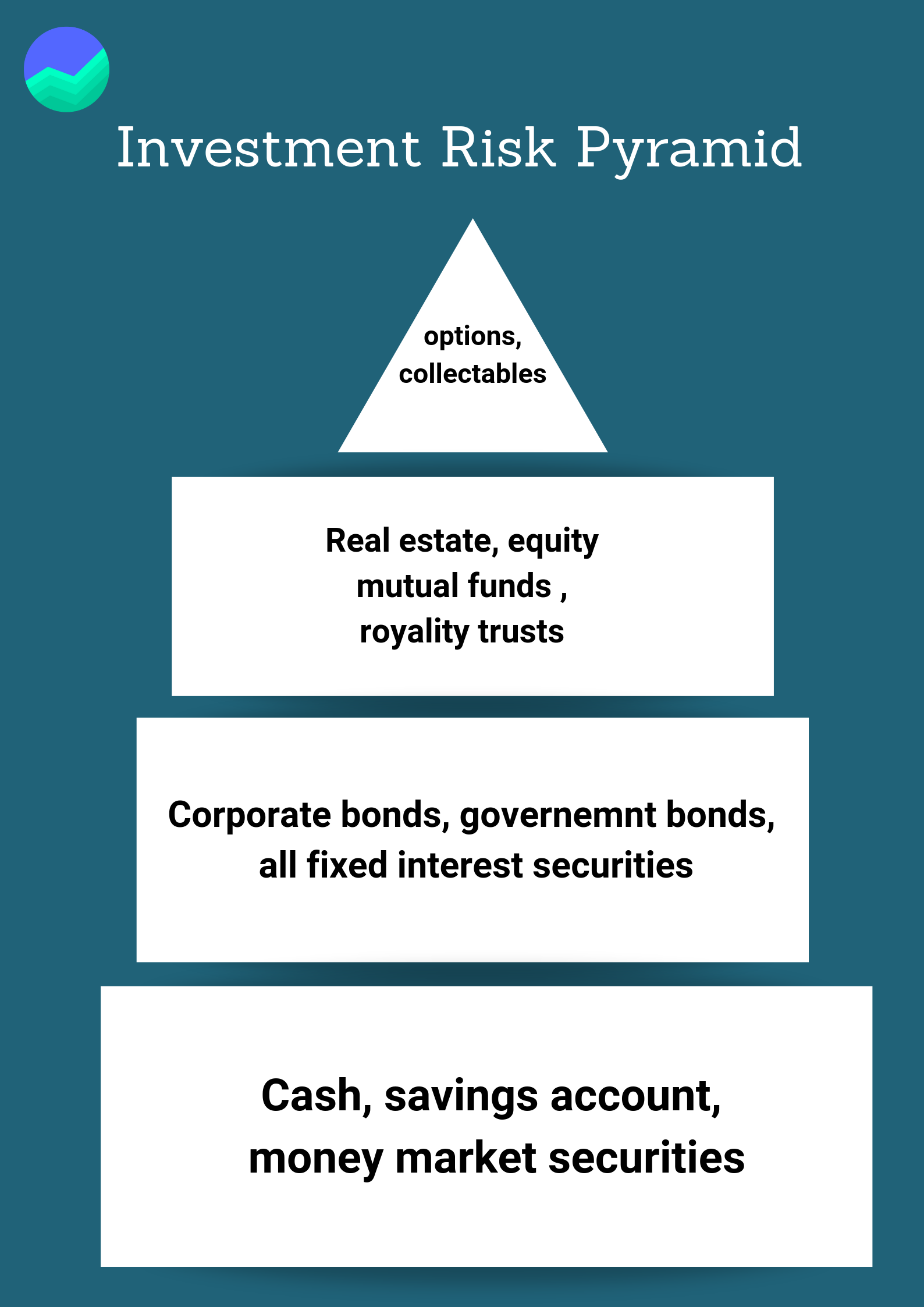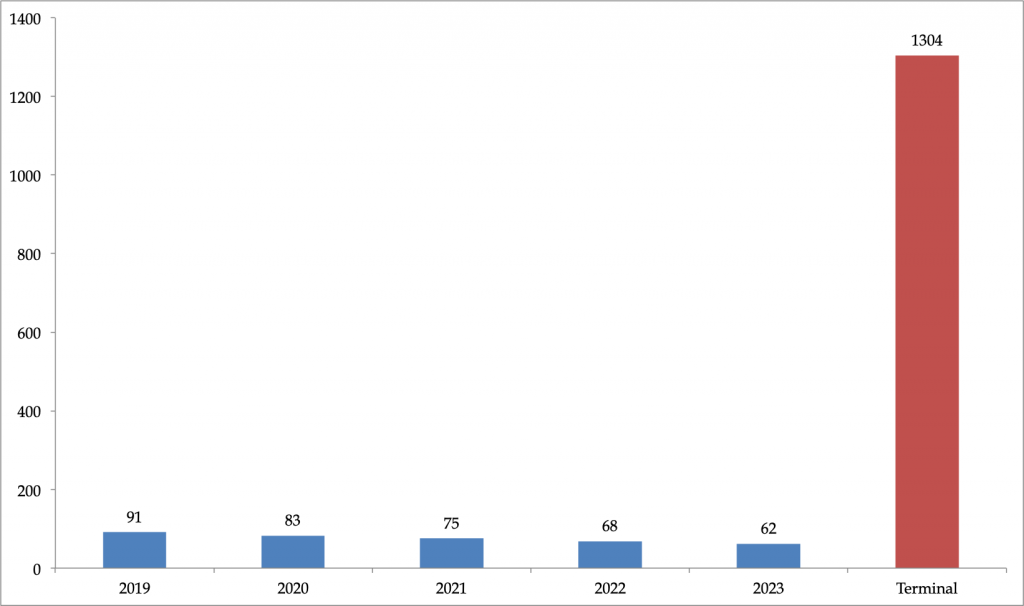Intrinsic value is the anticipated or calculated value of a company, stock, currency or product determined through fundamental analysis. It includes tangible and intangible factors. Intrinsic value is also called the real value and may or may not be the same as the current market value.

It is also referred to as the price a rational investor is willing to pay for an investment, given its level of risk.

## Basic Formula

The fundamental or the intrinsic value of a business or any investment asset is generally considered as the present value of all future cash flows discounted at an appropriate discount rate.

Thus, the most “standard” approach is similar to the net present value formula:

Where the symbols have the usual meaning shown below –

• NPV = Net Present Value
• CFi = Net cash flow for the ith period (for the first cash flow, i = 0)
• r = interest rate
• n = number of periods

## Breaking Down the Intrinsic ValueThe intrinsic value can be computed by value investors using fundamental analysis. In this method, an analyst has to look at both the qualitative factors and quantitative factors.

The qualitative factors include the business model, governance, and market factors, whereas the quantitative factors such as financial statement analysis/ The computed intrinsic value is then compared with the market value to determine if the asset is overvalued or undervalued.

Stocks that were least affected by the recession in 2008

## Risk Adjusting the Intrinsic Value

The risk of adjusting the cash flow is subjective. It is a combination of both art and science. There are two primary methods –

### 1. Discount Rate

Under this approach, the analyst generally uses a company’s weighted average cost of capital.

The weighted average cost of capital usually includes the risk-free rate (derived from the government bond yield) along with a premium based on the volatility of the stock multiplied by an equity risk premium.The approach is based on the fundamental theory that if a stock is more volatile, it is a riskier investment and an investor should get better returns.

Thus, in this situation, a higher discount rate is used, and it reduces the cash flow value that is expected in the future.

### 2. Certainty Factor

In this method, a certainty factor, or probability is assigned to each cash flow or multiplied against the entire net present value (NPV).

This method is a means of discounting the investment. In this method, the risk-free rate is used as the discount rate as the cash flows are risk adjusted.

For example, the cash flow from a government bond comes with a 100% certainty. Thus, the discount rate would be 7 percent.

Learn About Margin of Safety: Rule No.1 of Stock Market Investing!

Therefore, the discount rate is equal to the yield rate. Now, assume cash flow from a high growth company that has 50% probability factor assigned, the same discount rate can be used as the risk related to the high-risk asset (the high growth company in this case) is already factored in with the probability number.

### Challenges With Intrinsic Value

One of the difficulties with the value is that the method of intrinsic computing is a very subjective exercise. The technique involves numerous assumptions to project the cash flow.

Thus, the final net present value is sensitive to changes in these assumptions.

Another challenge is that while computing the weighted average cost of capital, the factors such as beta, market risk premium, etc. can be calculated differently. Also, the probability factor that is used is subjective.Lastly, by definition, the future is uncertain. Thus, while using the method, different investors can arrive at different values for the same asset. This difference is because everyone has a different way of looking at the future. Moreover, there is no way to say which number is accurate.

### Method of Valuation

While valuing a company as a going concern, there are three main methods used by industry practitioners:

Comparable company analysis, and

### Precedent Transactions

DCF analysis

Let us see each of the methods briefly:

## Method 1: Comparable Analysis

The method of comparative analysis is also known as trading multiples or peer group analysis or equity comps or public market multiples.

The method employs the technique of relative valuation in which an analyst compares the business (or asset) to be valued to other similar companies by studying trading multiples such as P/E, EV/EBITDA, or different other ratios.

The method provides an observable value for the business based on what other companies are worth.

Example, if a company A trades at 10x P/E ratio and company B has earnings of Rs. 2 per share, the value of each stock of company B is worth at Rs 20 per share (assuming the companies are entirely comparable).

## Method 2: Precedent Transactions

The method of precedent transactions is similar to relative valuation in which an analyst compares the company to be valued to other businesses that have been recently sold or acquired and belongs to the same industry.

These transactions are put to use to assess the value of the company.

## Method 3: DCF Analysis

DCF also is known as the Discounted Cash Flow (DCF) method is the most used approach to arrive at the intrinsic value. In this method, the analyst forecasts the future cash flow of the business and discount it to present value by using the firm’s Weighted Average Cost of Captial (WACC).

Let us now see an example to understand how fair value is determined with the help of the DCF method.

Assume you are evaluating a company that has the following cash flow.

Cash flow for the first five years starting 2019 – Rs 100
Discount rate – 10%
Terminal Growth rate – 5%
The present estimation of the cash flow generated in 2019 –

= CF / (1+r)^n

= 100/ (1+10%)^1

Thus, the present value is Rs 91

Terminal value is computed as perpetual growth. Formula is –

= {CF*(1+growth rate)}/(discount rate – growth rate)

Thus, the terminal value is

= {100*(1+5%)}/(10%-5%)

= Rs 2100

The present worth of the terminal value is calculated using the method shown above.

So, the present value of all future cash flows is shown as –

The present value of future cash flow (including terminal value)Thus, the fair value of the company is Rs 1683 (addition of the present value of all future cash flows).

## In Conclusion

To conclude, intrinsic value is a critical element of assessing a stock for investment purpose.

There are multiple ways of evaluating the reasonable amount, and an investor should ensure that he/she employs the best method based on the sector and characteristics of the company which is being evaluated.

Disclaimer: The views expressed in this post are that of the author and not those of Groww